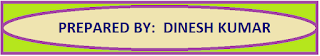### Resource Centre Mathematics

Resource Centre Mathematics Mathematics worksheet, mathematics basic points and formulas, mathematics lesson plan, mathematics multiple choice questions Workplace Dashboard CBSE Syllabus For Session 2023-24 For  :   Classes IX & X    |   Classes XI & XII Watch Videos on Maths Solutions CLASS IX MATHEMATICS FORMULAS &  BASIC CONCEPTS

### Math Assignment Ch-9 Class X | Application of Trigonometry

Maths Assignment Class 10th Chapter 9
Application of Trigonometry
Maths Assignment for CBSE Class 10th Chapter 9 Application of Trigonometry, Extra questions based on the topic Height and distance or application of trigonometry

 Mathematics Assignment Class 10 Chapter 9   Application of Trigonometry Question 1 From the window 15 m high above the ground in a street, the angles of elevation and depression of the top and foot of another house on the opposite side of the street are 30o and 45o respectively. Find the height of the opposite house.              ( Take √3 = 1.732)  [Answer 23.36] Question 2 The angle of elevation of an aeroplane from a point on the ground is 60o. After a flight of 30 seconds, the angle of elevation changes to 30o. If the plane is flying at a constant height of 3600√3 m, then find the speed of the plane in km/hour.    [Answer 864] km/h Question 3 The angle of elevation of an aeroplane from a point on the ground is 45o. After flying for 15 seconds, the angle of elevation changes to 30o. If the aeroplane is flying at a constant height of 2500 m, then find the average speed of the aeroplane.                       [Answer  439.2 km/h] Question 4 A man standing on the deck of a ship, which is 10 m above the water level, observes the angle of elevation of the top of a hill as 60o and the angle of depression of the base of the hill as 30o. Find the distance of the hill from the ship and the height of the hill.  [Answer  10√3 m,   40 m] Question 5Two ships are approaching a light house from opposite direction, the angle of depression of the two ships from the top of light house are 30o and 45o. If the distance between the two ships is 100 m, find the height of the light house.  ( Take √3 = 1.732)   [Answer  36.60 m] Question 6 The angle of elevation of the cloud from a point 200 m above the lake is 30o and the angle of depression of its reflection in the lake is 60o. Find the height of the cloud from the level of the lake . [Answer  400m] Question 7: From the top of the tower, the angles of depression of two objects on the same side of the tower are found to be 𝞪 and 𝛃 (𝞪 > 𝛃). If the distance between the objects is K meters  apart, then show that the height h of the tower is  given by Question 8 From the top of the tower 60 m high, the angles of depression of the top and bottom of a pole are observed to be 45o and 60o respectively. Find the height of the pole.  [Answer 20(3 - √3)m ] Question 9 A man in a boat, rowing away from a light house 100 m high, takes 2 minutes to change the angle of elevation of the top of the light house from 60o to 45o. Find the speed of the boat. [Answer  50(3-√3)/3 m/min] Question 10 The angle of elevation of a cloud from a point 60m above a lake is 30o and the angle of depression of the reflection of cloud in the lake is 60o. Find the height of the cloud. Question 11 Two equal poles on either side of the road 180m wide. The angles of elevation of the top of the pillars are 60o and 30o at a point between the pillars on the roadway. Find the height of the pillars and the position of the point              [Ans: 77.94m,  135m] Question 12 An aeroplane when flying at a height of 5000m above the ground passes vertically above another plane at an instant when the angle of elevation of the two planes from the same point on the ground are 60o and 45o respectively. Find the vertical distance between the two planes at that instant.                  Take  √3 = 1.73                     [Ans. 2116.7 ] Question 13 From the top of a light house, the angles of depression of two ships on the opposite sides of it are observed to be 30o and 60o. If the height of the light house be 150m, then find the distances between two ships.                           [Ans  200√3] Question 14 An aeroplane flying horizontally 1000m above the ground is observed at an angle of elevation 60o from the point on the ground. After a flight of 10 seconds, the angle of elevation at the point of observation changes to 30o. Find the speed of the plane in m/s.  Take  √3 = 1.73                                                              [Ans : 115.33 m/s.] Question 15 A man on the top of the vertical tower observes a car moving at a uniform speed coming directly towards it. If it takes 12 minutes for the angle of depression to change from 30o to 45o, how soon after this will the car reach the observation tower ? Take  √3 = 1.73                                                                  [Ans 16.38 minutes] Question 16 As observed from the top of a light house, 100m high above the see level, the angles of depression of a ship, sailing directly towards it ,changes from 30o to 60o. Find the distance travelled by the ship during the period of observation.   [Ans: 115.3 m] Question 17 The angles of elevation of the tower from the two points on the ground at distances a and b from the foot of the tower are complementary. Prove that the height of the tower is  √ab

##Download Pdf Form of Mathematics Assignments

1.Please make there pdf to make our work easy and your give question are very good